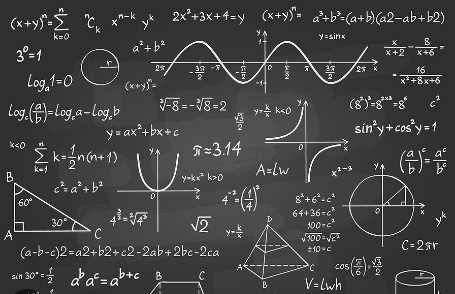# blog

Home / DeveloperSection / Blogs / What level of math is required for Machine learning?# What level of math is required for Machine learning?

75 19-Jan-2023

Machine learning is one of the most exciting and rapidly growing fields in technology today. It is used in a wide range of applications, from self-driving cars to image recognition to natural language processing. But what level of math is required to understand and work with machine learning algorithms?

It's also worth noting that machine learning is a highly interdisciplinary field that draws from a variety of other disciplines, such as statistics, physics, and electrical engineering. Having a broad understanding of these other fields can help you better understand the underlying principles of machine learning and how they can be applied in different contexts.

What level of math is required for Machine learning?

The short answer is that some basic mathematical knowledge is necessary to understand the concepts behind machine learning. However, the level of math required varies depending on the specific area of machine learning you want to work in.

For example, if you want to work on supervised learning problems, such as regression or classification, a good understanding of linear algebra and probability is essential. Linear algebra is used to manipulate matrices and vectors, which are fundamental data structures in machine learning. Probability is used to model uncertain events, which is crucial for understanding how machine learning algorithms make predictions.

If you want to work on unsupervised learning problems, such as clustering or dimensionality reduction, knowledge of linear algebra and basic statistics is important. Clustering algorithms, for example, use linear algebra to group similar data points together, while dimensionality reduction algorithms use statistics to identify the most important features in a dataset.

Deep Learning

Deep learning, which is a subset of machine learning that is used for image and speech recognition, requires a good understanding of linear algebra, probability, and calculus. The algorithms used in deep learning, such as neural networks, require the ability to perform complex matrix operations and optimize large sets of parameters, which requires calculus.

In addition to these specific mathematical skills, it is also important to have a good understanding of computer science concepts, such as algorithms and data structures. This will help you understand how machine learning algorithms are implemented and how to optimize their performance.

Additionally, having a strong background in programming is also crucial for working in machine learning. The ability to code and implement machine learning algorithms is essential for building and testing models, as well as for deploying them in real-world applications.

Overall, while some mathematical knowledge is required to understand machine learning, the level of math needed varies depending on the specific area of machine learning you want to work in. If you are interested in machine learning and have a strong background in math, it is definitely worth pursuing further study in the field. There are many resources available, including online courses, books, and tutorials, to help you gain the knowledge and skills you need to succeed in machine learning.

Conclusion

In conclusion, Machine learning is a dynamic field that requires a solid foundation of mathematical and computer science concepts. While the level of math required varies depending on the specific area of machine learning you want to work in, a good understanding of linear algebra, probability, and calculus is essential for deep learning. The field is growing exponentially, and there is a high demand for professionals with the knowledge and skills to work in this exciting field.

Also, while a solid foundation in math is essential for understanding the concepts behind machine learning, it is also important to have a broad understanding of other related disciplines and to be proficient in programming. By cultivating a well-rounded skill set, you will be well-prepared to succeed in the exciting and rapidly growing field of machine learning.#### HARIDHA P

A passionate writer, blogger, language trainer, co-author of the book 'Irenic' and an enthusiastic learner. Interest includes travelling places and exploring.